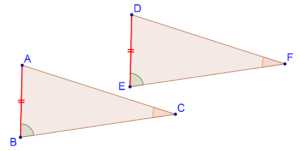# Is There an AAS Criterion?

Go back to  'Triangles-Quadrilaterals'

We have proven the ASA criterion when two angles and the included side (the side between the two angles) of one triangle are (correspondingly) equal to two angles and the included side of another triangle. But will the ASA criterion hold if the equal sides are non-included? In other words, is there something like an AAS criterion?

The answer, as you may be able to anticipate, is yes! If two angles of a triangle are equal to two angles of another, then their third angles are automatically equal, since the sum of angles in any triangle must be a constant (180°).

Consider the following figure:In ∆ABC and ∆DEF, we are given that

1. ∠B = ∠E

2. ∠C = ∠F

3. AB = DE (a non-included side)

Can we say that the two triangles are congruent? Yes, because ∠A and ∠D must also be equal, and thus our ASA criterion applies as we discussed earlier.

To summarize: when we have to apply the ASA criterion for congruence between two triangles, we only need to check that:

1. Two pairs of angles should be (correspondingly) equal.

2. Any pair of sides should be (correspondingly) equal.